# Frank Solutions for Chapter 9 A Ammonia Class 10 Chemistry ICSE

1. How ammonia occurs in nature?

Ammonia is found both in free state and in combined state. In free state, it is formed in traces amount by decaying urine and other organic matter.
In combined state, ammonia is found as ammonium salts mainly as ammonium chloride and ammonium sulphate.

2. (a) Give the formula of (i) Liquid ammonia (ii) Liquor ammonia
(b) What is liquor ammonia fortis?

(a) (i) Liquid ammonia - Compressed ammonia gas at 6 atmospheric pressure. Chemical formula - NH3
(ii) Liquor ammonia - It is saturated solution of ammonia in water. It is very dilute solution of ammonium hydroxide (NH4OH).
(b) A saturated solution of ammonia in water is called liquor ammonia Fortis.

3. (i) How is ammonia gas prepared in laboratory starting from NH4Cl? State the conditions and balanced equation for the preparation.
(ii) How is gas collected in the gas jar?
(iii) Name the substance used for drying ammonia gas? Why cannot substances such as conc.H2SO4, anhydrous calcium chloride and phosphorus pentoxide be used for drying
ammonia gas?

(i) Ammonium chloride is heated with calcium hydroxide (an alkali), in ratio of 2: 3 by weight, to produce ammonia.
Reactants should be dry and in grounded state. The reactants are heated to get the products.
Balanced reaction:Key: Laboratory Preparation of Ammonia
(ii) Ammonia gas is collected in an inverted dry gas jar by the downward displacement of air.
(iii) The substance used for drying ammonia gas is quicklime(calcium oxide).Concentrated sulphuric acid, anhydrous calcium chloride and phosphorus pentoxide cannot be used for drying ammonia gas because ammonia being basic reacts with them, and the following reaction takes place
Conc. H2SO4  reacts with ammonia to form ammonium sulphate.
2NH3  + H2SO4 (conc.) (NH4)2SO4
Phosphorus pentoxide reacts with ammonia to form ammonium phosphate.
6 NH3  + P2O5  + 3H2O ⟶ 2(NH4)3PO4
Anhydrous calcium chloride combines with ammonia to form addition compound.

4NH3 + CaCl2  ⟶ CaCl2 .4NH3

4 . Name the following
(i) Gas obtained by treating chlorine in excess of ammonia.
(ii) Solid obtained by passing ammonia over heated copper oxide
(iii) Products obtained by burning ammonia in oxygen.
(iv) Substance used to dry ammonia
(v) Liquid when added to metallic nitride that yield ammonia.
(vi) Indicator that turns deep pink when treated with NH4OH.
(vii) Products obtained by treating with excess chlorine.
(viii) Basic gas that is used as a refrigerant.
(ix) Solution used to remove fat grease.
(x) Salt known as ammoniac.
(xi) Salt used to clean metal surface before soldering, tinning, etc.
(xii) Ammonium salt used in explosive.
(xiii) An acidic gas which reacts with a basic gas liberating neutral gas.
(xiv) A nitride of divalent metal which reacts with hot water producing ammonia.

(i) Nitrogen gas
(ii) Copper
(iii) Nitrogen and water vapour
(iv) Quick lime (calcium oxide)
(v) Warm water
(vi) Phenolphthalein
(vii) Nitrogen trichloride and hydrogen trichloride
(viii) Liquid ammonia
(ix) Ammonia solution
(x) Ammonium chloride
(xi) Ammonium chloride
(xii) Ammonium nitrate5.(a) Name the process used to manufacture ammonia from its elements.
(b) Under what conditions do the reactants combine to form ammonia? Give a balanced equation for the reaction.
(c) In what ratio by volume, are the above reactants used.
(d) State one possible source of each reactant used in the process.
(e) What is the function of:
(i) finely divided iron
(ii) Molybdenum in the above process
(f) Mention two possible ways by which ammonia produced is removed from unchanged gases.

(a) Haber's Process

(b) The reactants nitrogen and hydrogen combine at low temperature and high pressure in presence of catalyst to form maximum yield of ammonia. The balanced equation for the reaction is:(c) The mixture of dry nitrogen and dry hydrogen is mixed in the ratio of 1:3 by volume to produce ammonia.
(d) Source of hydrogen: Hydrogen is generally obtained from water gas by Bosch process.
Source of nitrogen: Nitrogen is obtained from air by its liquefaction and fractional distillation.
(e) (i) Finely divided iron acts as catalyst which alters the rate of reaction, but itself does not undergo any chemical change.
(ii) Molybdenum acts as promoter which increase the efficiency of the catalyst.

(f) The gases leaving the catalyst chamber are cooled by passing through condensing pipes where ammonia gets liquefied and is collected in receiver.
Key: Haber's Process

6. How will you demonstrate the solubility of ammonia in water? Explain.

Ammonia is highly soluble in water. This can be demonstrated by Fountain experiment.

• We take a round bottom flask filled with ammonia gas.
• The flask is closed with a two holed rubber stopper, one for the jet tube and the other for a dropper filled with water.
• The flask is fixed to the stand in an inverted position.
• The free end of the jet tube is dipped into a beaker containing red litmus solution.
• When the bulb of dropper is pressed, it is observed that red litmus solution rises up and strikes the wall of the flask and spreads in form of fountain, which is blue in colour.

7. Write the equation for the action of heat on:
(i) Ammonium chloride
(ii) Ammonium nitrate
State whether each reaction is an example of thermal dissociation or thermal decomposition.

(i) Action of heat on ammonium chloride is reversible. When heated, it decomposes to form ammonia and hydrogen chloride. When the products are cooled, they recombine to form ammonium chloride.This is thermal dissociation reaction.
(ii) Ammonium nitrate on heating completely disappears, escaping in the form of water vapour and nitrous oxide.This is thermal dissociation reaction.

8. List the properties of ammonia that make it
(i) A good refrigerant
(ii) A cleaning agent
(iii) As a source of hydrogen

(i) Liquid ammonia is used as refrigerant as:

• It is highly volatile
• It has high specific latent heat of vaporization. 1 mole (17g) of liquid ammonia vaporises by absorbing 5.7 kcals of heat from the surroundings, which is there by cooled.
• It easily liquefies under pressure at room temperature.
(ii) Ammonia emulsifies fats and grease. Thus it is used to clean oils, fats and body grease etc. from clothes.
(iii) Liquid hydrogen is dangerous to transport as it is highly combustible. Thus, hydrogen is converted to liquid ammonia and transported in cylinders. Later it is catalytically converted to hydrogen.
Key: Uses of ammonia

9. (a) Give three uses of ammonium chloride.
(b) Why is ammonium hydroxide used in qualitative analysis? Give two equations to justify your answer.

(a) Three uses of ammonium chloride :

1. For cleaning metal surfaces before soldering, tinning, etc.
2. In Leclanche cell and in dry cell.
3. In medicine and dyeing.
(b) Ammonium hydroxide precipitates hydroxides of metals when it is added to aqueous solution of their salts. The precipitate of metal hydroxides differ in colour and solubility and hence ammonium hydroxide is used in qualitative analysis.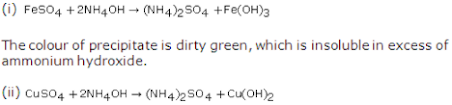The colour of precipitate is bluish white, which is soluble in excess of ammonium hydroxide.

10. Distinguish between liquid ammonia and liquid ammonia fortis.

 Liquid ammonia Liquid ammonia fortis 1. It is obtained by compressing ammonia gas at 6 atmospheric pressure. 1. It is a saturated solution of ammonia in water. 2. It is used as refrigerant 2. It is used as a laboratory agent.

11. Give three reactions to show reducing property of ammonia.

Ammonia is strong reducing agent. It is oxidised to nitrogen by removal of hydrogen, when it react with oxidizing agent.

12. Draw the structure of ammonia and give the reason for alkaline nature of aqueous ammonia.

SolutionThe presence of lone pair of electrons on the nitrogen atom makes ammonia basic in nature in its aqueous solution. Aqueous solution of ammonia dissociates partially to produce hydroxyl ion.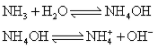Key: Aqueous solution of ammonia is alkaline in nature.

13. How is an aqueous solution of ammonia prepared? Stage two advantages of method used.

An aqueous solution of ammonia is prepared by dissolving ammonia in water. Ammonia is highly soluble in water.The funnel arrangement is used to prepare aqueous solution of ammonia. In this method, ammonia gas is introduced through a funnel tube where the rate of dissolution of ammonia is faster than it is produced. This creates a partial vacuum, in delivery tube, resulting in low pressure. Thus, water is forced in to equalise the pressure. To overcome this problem of back suction, the funnel is attached to outer end of the delivery tube and mouth of funnel just dips into the water in the trough.
(i) Prevents back suction of water into the flask.
(ii) Provides a larger surface area for the absorption of the gas.

Key: Ammonia is highly soluble in water.

14. (a) Name two fertilizers manufactured from ammonia.
(b) Write an equation for the reaction to prepare one fertilizer from ammonia.

(a) Two fertilizers manufactured from ammonia : Urea, ammonium sulphate, ammonium nitrate.
(b) Fertilizer from ammonia : UreaKey : Ammonia is used in manufacture of fertilizer.

15. Explain the following:
(i) The source of heat is removed after sometime in Haber's process.
(ii) Ammonium nitrate is not used in the laboratory preparation of NH3.
(iii) Ca(OH)2 is preferred over NaOH in the preparation of ammonia.
(iv) Liquid NH3 has no action on litmus, while liquor ammonia has an effect.
(v) Dry N2 and H2 must be used in the Haber's process.
(vi) A promoter is added along with the catalyst in the Haber's process.
(vii) Aqueous ammonia conducts electricity.
(viii) Ammonia cannot be collected over water.
(ix) Ammonia is present in sewage water.
(x) Ammonia solution is used in the laboratory to identify metal ions.

(i) Once the reaction starts, further heating is not required because the reaction is exothermic. The heat evolved is sufficient to maintain the optimum temperature.
(ii) Ammonium nitrate is explosive in nature and it decomposes to give nitrous oxide and water. Thus, it is not used in the laboratory preparation of ammonia.
(iii) Calcium hydroxide is preferred as it is cheap and not deliquescent like NaOH or KOH.
(iv) Liquid ammonia is a polar covalent compound and neutral to litmus. Liquor ammonia is solution of ammonia in water, which is weakly basic in nature. It turns red litmus blue.
(v) Presence of moisture may result in formation of ammonium hydroxide. Thus dry N2  and H2  must be used in the Haber's process.
(vi) A promoter is a substance that is added to increase the efficiency of a catalyst.
(vii) Aqueous solution of ammonia that is ammonium hydroxide dissociates partially to hydroxyl ions. Thus, it conducts electricity.(viii) Ammonia is highly soluble gas in water and so cannot be collected over water.
(ix) Ammonia is formed by decaying urine and other organic matter. Thus it is present in sewage water.
(x) Ammonium hydroxide precipitates hydroxides of metals, which differ in colour and solubility in excess of ammonium hydroxide. Thus it is used in identification of metal ions.

16. Give balanced reactions for the following conversions:
(i) Ammonia to nitrogen using an acidic gas.
(ii) Ammonia to nitrogen using copper oxide.
(iii) Ammonia to nitrogen using oxygen.
(iv) Ammonia to nitrogen using trichloride.
(v) Ammonia to ammonium sulphate.
(vi) Ammonia solution to an amphoteric hydroxide.
(vii) Ammonia to ammonium carbonate
(viii) Copper oxide to copper.

17. What do you observe when
(i) Excess ammonia is mixed with chlorine.
(ii) Ammonia in excess is mixed with chlorine.
(iii) Filter paper dipped in colourless phenolphthalein is introduced into ammonia.
(iv) Ammonia is passed over heated lead oxide.
(v) Ammonium solution is added to ferric chloride solution.
(vi) Ammonia solution is added drop by drop and then in excess to aqueous copper sulphate solution.
(vii) Ammonia comes in contact with the eyes of a person.

18. Give balanced equation to prove that ammonia
(a) Is alkaline in nature
(b) Has the element hydrogen in it.
(c) Has the element nitrogen in it.

19. Differentiate between:
(i) Action on indicators or dry ammonia gas and aqueous ammonia
(ii) Reaction of excess ammonia with chlorine and NH with excess chlorine
(iii) Aqueous ferrous and ferric sulphate solution

(i) Difference between Dry Ammonia and Aqueous Ammonia

 Dry ammonia Aqueous ammonia Dry ammonia is neutral to indicators. Aqueous ammonia shows alkaline or basic behaviour with indicators. It turns red litmus blue.

(ii) Difference between Reaction of excess ammonia with chlorine and Ammonia with excess chlorine

 Reaction of excess ammonia with chlorine Reaction of ammonia with excess chlorine 1. It forms ammonium chloride and nitrogen. It forms nitrogen trichloride and hydrogen chloride 2. 8NH3(excess) + 3Cl2 → N2 + 6NH4Cl NH3 + 3Cl2(excesses) → NCl3 + 3HCl 3. The yellow green colour of chlorine gas disappears and white ammonium chloride is formed . Nitrogen trichloride is a yellow coloured highly explosive liquid.

(iii) Difference between Aqueous ferrous and ferric sulphate solution

 Aqueous ferrous Ferric sulphate solution When ammonium solution is added to aqueous solution of ferrous sulphate a dirty green precipitate of ferrous hydroxide is obtained which is insoluble in excess of ammonium solution.Hydroxide.FeSO4 + 2NH4OH → (NH4)2SO4 + Fe(OH)2 On adding ammonium solution to ferric sulphate solution we get ammonium sulphate and reddish brown precipitate of ferric hydroxide which is insoluble in excess of ammonium solution.Fe2(SO4)3 + 6NH4OH → 3(NH4)2SO4 + 2Fe(OH)3

20. (a) Give one use each of four different compounds of ammonia.
(b) Describe two tests to identify ammonia and ammonium ions in an aqueous solution.

(a) (i) Use of Ammonium Chloride
Used in Leclanche cell and dry cell
(ii) Use of Ammonium Sulphate
Used as a fertilizer
(iii) use of Ammonium nitrate
Used in fireworks
(i) Use of Ammonium Carbonate
Used in baking powder

(b) Test of ammonia and ammonium ions:

1. Ammonia gas has a characteristic pungent smell
2. A glass rod dipped in concentrated hydrochloric acid and is introduced into the gas produces thick white fumes of ammonium chloride.

21. Ammonia reacts with a monobasic acid (A) to form a salt (B). B decompose at about 250oC to give two products (C) and (D), leaving no residue. The oxide (D) is a liquid at room temperature and neutral to moist litmus, while gas (C) is a neutral oxide. Identify A, B, C, D. Write the balanced equation involved the above process.

22. Describe all what you will observe and write chemical equations, when limited amount of ammonia gas is passed through the following aqueous solutions:
(i) ZnCl2
(ii) FeSO4
(iii) FeCl3
(iv) Pb(NO3)2
(v) CuSO4
(vi) CrCl3

(i) Ammonia gas on reacting with aqueous solution of zinc chloride produces white gelatine like precipitate of zinc hydroxide.(ii) Ammonia gas on reacting with aqueous solution of ferrous sulphate produces dirty green precipitate of ferrous hydroxide.(iii) Ammonia gas on reacting with aqueous solution of ferric chloride produces reddish brown precipitate of ferric hydroxide.(iv) Ammonia gas on reacting with aqueous solution of lead nitrate produces white precipitate of lead hydroxide.(v) Ammonia gas on reacting with aqueous solution of copper sulphate produces bluish white precipitate of cupric hydroxide.(vi) Ammonia gas on reacting with aqueous solution of Chromium chloride produces green precipitate of chromium tri hydroxide.23. What are chlorofluorocarbons, why they become popular as substitute of ammonia as refrigerant?

A chlorofluorocarbon (CFC) is an organic compound which contains carbon, chlorine and fluorine produced as a volatile derivative of methane and ethane.
Pure ammonia gas is highly toxic to humans and would pose a threat if the refrigerator were to leak. Hence, CFCs became popular as a substitute of ammonia as a refrigerant.

24. Give two examples of suitable alternatives of CFCs which can be used as refrigerant and are non-ozone deflecting?

(i) Hydrochlorofluorocarbon (CF3CHCl2)
(ii) Hyrofluorocarbon (HFC)

25. Choose the correct answer from the options given below :
(i) Ammonia is produced when ammonium chloride is heated with
(a) Potassium nitrate
(b) Slacked lime
(c) Quick lime
(d) Sodium nitrate
(b) Slacked lime

(ii) Ammonia reacts with excess chlorine to form
(a) NH4Cl
(b) NH3, NCl3, HCl
(c) N2, NH4Cl
(d) NCl3, HCl
(d) NCl3, HCl

(iii) Nessler's reagent is an alkaline solution of
(a) HgI2
(b) K2HgI4
(c) HgNH2I
(d) NH2HgO, HgI
(b) K2HgI4

(iv) Ammonia is commercially prepared by
(a) Haber's process
(b) Ostwald process
(c) Contact process
(a) Haber's process

(v) The other products obtained along with water where NH3 is passed over heated CuO are
(a) Cu(NH2)and H2
(b) [Cu(NH3)4]2+ and O2
(c) Cu and N2
(d) None of these
(c) Cu and N2

(vi) Liquid ammonia is used in refrigeration because of its
(a) High dipole moment
(b) Baricity
(c) Stability
(d) High heat of vaporization
(d) High heat of vaporisation

(vii) In the Haber's process for the manufacture of NH3, iron is used as catalyst and molybedenum as promoter. The function of promoter is
(a) To Increase the rate of combination of gases
(b) To raise the activation energy of the reaction
(c) To increase the activity of the catalyst
(d) To increase the yield of ammonia.
(c) To increase the activity of the catalyst

26. Name a chloride which is soluble in excess of ammonium hydroxide.

Silver chloride

27. Ammonia cannot be collected over water. Give reasons.

Ammonia is highly soluble gas in water and so cannot be collected over water.

28. Pick the odd number out from the list, giving reasons for your answer:
Ammonia, sulphur dioxide, hydrogen chloride, carbon dioxide

Ammonia is the odd one out.
Ammonia forms weakly basic solution when dissolved in water.
The others give acidic solution when dissolved in water.

29. Write balanced equation for the preparation of ammonia for ammonium chloride.

Ammonium chloride on heating with an alkali produces ammonia with other products.30. Give two large scale use of ammonia.

1. Ammonia is used in the manufacture of fertilisers such as ammonium sulphate, ammonium nitrate, etc.
2. It is used in the industrial preparation of nitric acid by Ostwald's process.

31. State what will you observe when ammonium hydroxide solution is added to copper sulphate in excess.

Initially when ammonium solution is added to aqueous solution of copper sulphate a bluish precipitate of copper hydroxide is obtained which dissolves in excess of ammonium hydroxide.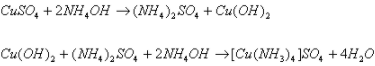32. Complete and balance the equation : CuO (heated) + NH3 ⟶

33. Explain, why ammonia is evolved when water is added to the product formed, when magnesium is burned in air.

Magnesium on burning in air produces magnesium nitride.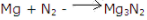When this magnesium nitride comes in contact with water ammonia gas is released.Key : Ammonia is formed from metal nitrides

34. Copy and complete the following table :

 Name of the products Name of the catalyst Approximate temperature Approximate pressure Haber’s Process

 Name of the products Name of the catalyst Approximate temperature Approximate pressure Haber’s Process Ammonia Iron 450 - 500°C 200-900 atmospheres

35. Write a balanced equation for the laboratory preparation of ammonia from ammonium chloride.

Ammonium chloride on heating with an alkali produces ammonia with other products.36. When ammonium hydroxide is added to a solution B, a pale blue precipitate is formed. The pale blue precipitate dissolves in excess of ammonium hydroxide to give an inky blue solution. What is the cation (positive ion) present in solution B? What is the probable colour of solution B?

The cation is Cu2+ ion. Solution B is copper sulphate. It is bright blue in colour.

37. When an ammonium salt is warmed with sodium hydroxide solution, ammonia gas is evolved. State three ways by which you can identify this gas.

Three ways to identify ammonia gas:

1. It is a pungent smell gas.
2. It gives white precipitate when bubbles through a solution of lead nitrate.
3. It gives a brown colour or precipitate when treated with Nessler's reagent.

38. Copy and complete the following equations :
(i) Mg3N2 + 6H2O →
(ii) 2NH3 + 3CuO →
(iii) 8NH3 + 3Cl2
(iv) 4NH3 + 5O2

39. Which property of ammonia is illustrated by the reaction (3-ii) in the above equation?

This reaction shows that ammonia is a reducing agent.

40. Name the important process that starts with the reaction in (3-iv). Name the catalyst used.

This process is called as Ostwald's Process. The catalyst used is platinum.

41. During the laboratory preparation, how is ammonia dried and collected?

During laboratory preparation of ammonia, it is passed through a drying tower containing quicklime (calcium oxide).
Ammonia is collected in an inverted dry gas jar by the downward displacement of air.

42. Name the gas evolved when the mixture of calcium hydroxide and ammonium chloride is heated.

Ammonia gas

43. What do you observe when ammonia is bubbled through red litmus solution?

Ammonia forms ammonium hydroxide and turns red litmus blue as it is alkaline in nature.

44. Write an equation for the reaction, when ammonium chloride and sodium hydroxide solution are mixed and heated.

45. What is the purpose of Haber's process? Name the gaseous inputs in the Haber's process and state the ratio by volume in which the gases are mixed. What is done to increase the rate of the reaction in Haber's process? Give two different ways by which the product can be separated from the reactants.

Haber's process is used in industrial preparation of ammonia.
Gaseous inputs in Haber's process are dry nitrogen and dry hydrogen gas. They are mixed in the ratio of 1:3 by volume.
The following conditions favour maximum yield of ammonia:

• Low temperature
• High pressure
• Use of catalyst
The gases after reaction pass through condensing pipes of cooling chamber where ammonia gets liquefied and is collected in receiver.
Ammonia can also be collected by downward displacement of air.

46. Write equation for the following:
(i) Burning of ammonia in oxygen
(ii) Catalytic oxidation of ammonia
(iii) (a) Name the catalyst used in 1(ii)
(b) In the reaction referred to in 1(ii), the catalyst glows red hot. Why?
(c) What is the name of the industrial process which starts with the reaction referred to in 1 (ii)?

47. (i) How is ammonia soluble in water?
(ii) Give two reasons to show that the solution of ammonia in water contains hydroxyl ions.
(iii) Name a simple method you would employ to prepare ammonium salts in your laboratory.

(i) When ammonia dissolve in water, it form ammonium hydroxide which dissociates into NH4+ and OH ion. Therefore it become soluble in water.
(ii) (a) Aqueous solution of ammonia turns red litmus blue stating that it is having basic ions. (b) It precipitates hydroxides of metals.
(iii) An aqueous solution of ammonia i.e. ammonium hydroxide reacts with acid to produce ammonium salt and water.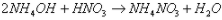48. State what would you observe when a piece of moist red litmus paper is placed in a gas jar of ammonia.

Dry ammonia are neutral to litmus. An aqueous solution of ammonia turns red litmus blue stating that it is basic in nature.

49. Ammonium salt decompose on heating. What other property do ammonium salts have in common?

Ammonium salts are used as fertilizers in fields.

50. Look at the following reaction :
A. Nitrogen + metal → compound X
B. X + water ⟶ ammonia + another compound
C. Ammonia + metal oxide ⟶ metal + water + N2
(i) One metal that can be used reaction in A is magnesium.
(a) Write the formula of the compound X, that is formed.
(b) Write the balanced equation for reaction B, where X is the compound formed.
(c) Which property of ammonia is demonstrated by reaction C ?

51. Industrially, ammonia is obtained by direct combination of nitrogen and hydrogen.
(i) Write balanced equation for the direct combination of nitrogen and hydrogen.
(ii) Which of the metals - iron, platinum, copper catalyse this direct combination?
(iii) Is the formation of ammonia promoted by high pressure or low pressure?

(i) The reactants nitrogen and hydrogen combine to form ammonia at low temperature, high pressure in presence of catalyst to for maximum yield of ammonia. the balanced equation for the reaction is :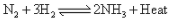(ii) Iron acts as catalyst.
(iii) Since the forward reaction occurs with decrease in volume, according to Le Chatelier's principle, high pressure favours the formation of ammonia.
Key : Haber's process

52. Is ammonia denser than air? Which property of ammonia is demonstrated by the Fountain experiment? Write the balanced equation for the reaction between ammonia and sulphuric acid.

Ammonia (vapour density (V.D) = 8.5) is less dense than air (vapour density (V.D.) = 14.4) and so is lighter than air.
Fountain experiment proves that ammonia is highly soluble in water.
The balanced equation for the reaction between ammonia and sulphuric acid is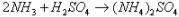53. Choose the correct word or phrase from the bracket to complete the following sentences:
(i) Heating ammonium chloride with sodium hydroxide produces ______ (ammonia, nitrogen).
(ii) Heating solution of ammonium chloride with sodium nitrite produces ______(ammonia, nitrogen).

(i) Ammonia
(ii) Nitrogen

54. Name the gas produced on warming ammonium sulphate with sodium hydroxide solution.

Ammonia

55. Write the equation for:
(i) The preparation of ammonia from ammonium chloride and calcium hydroxide.
(ii) The reaction of hydrogen chloride with ammonia.

56. What are the products formed when ammonia is oxidised with copper oxide?

57. What is the difference between the chemical nature of an aqueous solution of hydrogen chloride and an aqueous solution of ammonia?

Aqueous solution is acidic in nature due to presence of hydrogen ion.
Aqueous solution of ammonia is weakly basic in nature due to presence of hydroxyl ion.

58.  Write the equations for the action of heat on ammonium chloride and ammonium nitrate?

Action of heat on ammonium chloride is reversible. When heated, it decomposes to form ammonia and hydrogen chloride. When the products are cooled, they recombine to form ammonium chloride.59. State whether each of the above reactions is an example of thermal decomposition or thermal dissociation.

Thermal dissociation.

60. Write the equation for the formation of ammonia by the action of water on magnesium nitride.

61. How is ammonia collected?

Ammonia is collected by downward displacement of air.

62. Which compound is normally used as a drying agent for ammonia?

Quick lime (calcium oxide) is used as a drying agent for ammonia.

63. Write equation for the reaction of chlorine with excess of ammonia.

64. (i) Write the equation for the reaction in the Haber process, that forms ammonia.
(ii) State the purpose of liquefying ammonia produced in the process.

(i) The reactants nitrogen and hydrogen combine to form ammonia at low temperature, high pressure in presence of catalyst to for maximum yield of ammonia. The balanced equation for the reaction is :(ii) The gases which leave the catalyst chamber contain ammonia and unreacted nitrogen and hydrogen gas. The gases leaving the catalyst chamber are cooled by passing through condensing pipes where ammonia gets liquefied and is collected in receiver.

65. (i) Which feature of the ammonia molecule leads to the formation of the ammonium ion when ammonia dissolves in water?
(ii) Name the other ion formed when ammonia dissolves in water.
(iii) Give one test that can be used to detect the presence of the ion produced in (i) and (ii).

66. Write the equations for the following reactions, which result in the formation of ammonia:
(i) A mixture of ammonium chloride and slaked lime is heated.
(ii) Aluminium nitride and water.

(i) Ammonium chloride on heating with an slaked lime produces ammonia with other products.67. State what is observed when excess of ammonia is passed through an aqueous solution of lead nitrate?

Ammonia gas on reacting with aqueous solution of lead nitrate produces white precipitate of lead hydroxide, which is insoluble in excess of ammonium hydroxide.68. (a) Name the substance used for drying ammonia.
(b) Write an equation to illustrate the reducing nature of ammonia.
(c) With reference to Haber's process for the preparation of ammonia, write the equation and the conditions required.

69. You enter a laboratory after a class has completed the Fountain experiment. How will you be able to tell whether the gas used in experiment was hydrogen chloride or ammonia?

By the pungent smell of ammonia gas.

70. Write balanced chemical equations for a reaction in which ammonia is oxidised by the following:
(i) A metal oxide
(ii) A gas which is not oxygen

71. Why is ammonia not collected over water?

Ammonia is highly soluble in water and so it is not collected over water.

Ammonia can be obtained by adding water to:
(a) Ammonium chloride
(b) Ammonium nitrite
(c) Magnesium nitride
(d) Magnesium nitrate

(c) Magnesium nitride

73. Write the equation for the following :
Ammonium chloride is treated with sodium hydroxide.

74. State your observation for the following cases
(i) Ammonia gas is burnt in an atmosphere of oxygen in the absence of a catalyst
(ii) Glass rod dipped in ammonium hydroxide is brought near the mouth of the concentrated hydrochloric acid bottle

(i) Ammonia burns with a yellowish flame. It produces water vapour and nitrogen.
(ii) When ammonium hydroxide is brought near the mouth of concentrated hydrochloric acid, it produces dense white fumes of ammonium chloride.

75. The questions below are related to the manufacture of ammonia.
(i) Name the process
(ii) In what ratio must the reactants be taken
(iii) Name the catalyst used
(iv) Give the equation for the manufacture of ammonia
(v) Ammonia can act as a reducing agent write a relevant equation for such a reaction.

(i) Haber's process
(ii) 1 part of nitrogen and 3 parts of hydrogen
(iii) Finely divided iron (Fe)76. The diagram shows a simple arrangement of the fountain experiment:(i) Name the two gases you have studied which can be used in this experiment
(ii) What is the common properly demonstrated by this experiment

(i) Ammonia and hydrogen chloride gas.
(ii) High solubility of gases in water.

77. The diagram shows an experimental set-up for the laboratory preparation of a pungent smelling gas. The gas is alkaline in nature.(i) Nature the gas collected in the jar.
(ii) Write the balance equation for the above preparation.
(iii) How is the gas being collected?
(iv) Name the drying agent used.
(v) How will you find that the jar is full of gas?

(i) Ammonia
(ii)(iii) By downward displacement of air
(iv) Quicklime/CaO
(v) Bring moist red litmus paper to the mouth of the inverted jar; it immediately turns blue.
Or
Bring a glass rod dipped in hydrochloric acid to the mouth of the inverted jar. If it produces dense white fumes, then the jar is full of gas.

78. Copy and complete the following table relating to important industrial process.

 Name of the process Temperature Catalyst Equation for the catalyzed reaction Haber’s Process

 Name of the process Temperature Catalyst Equation for the catalyzed reaction Haber’s Process 450 - 500°C Finelydividediron (Fe)79. Give balanced equation of
(a) Reduction of hot copper (II) oxide to copper using ammonia gas
(b) Action of heat an a mixture of copper an nitric acid

80. (i) Name the other ion formed when ammonia dissolves in water
(ii) Given one test can be used to detect the presence of the ion produced

(i) Hydroxyl (OH-) ion other than ammonium ion
(ii) Red litmus turns blue, methyl orange turns yellow and phenolphthalein turns pink.

81.Give balanced chemical equations for each of the following :
(i) Lab preparation of ammonia using an ammonium salt
(ii) Reaction of ammonia with excess chlorine
(iii) Reaction of ammonia with sulphuric acid

(i) 2NH4Cl + Ca(OH)2 → CaCl+ 2H2O + 2NH3
(ii) NH3 + 3Cl2 → NCl3 + 3HCl
(NCl3: nitrogen trichloride)

(iii) 2NH3+H2SO4 → (NH4)2SO4
[(NH4)2SO4: ammonium sulphate]

82. Name the gas evolved when the following mixtures are heated:
(i) Calcium hydroxide and ammonium chloride
(ii) Sodium nitrate and ammonium chloride

(i) Ammonia
(ii) Nitrogen

83. Write balanced chemical equations for each of the following
(i) When excess of ammonia is treated with chlorine
(ii) An equation to illustrate the reducing nature of ammonia

(i) 8NH3 + 3Cl2 → N2 + 6NH4Cl
(ii) 3PbO + 2NH→3Pb + 3H2O + N2

84. Identify the substance underlined, in the following case:
(i) Cation that does not form a precipitate with ammonium hydroxide but forms one with sodium hydroxide.
(ii) A solid formed by reaction of two gases, one of which is acidic and the other basic in nature.

(i) Cation that does not form a precipitate with ammonium hydroxide but forms one with sodium hydroxide: Ca2+
(ii) A solid formed by a reaction of two gases, one of which is acidic and the other basic in nature: Salt

85. (i) Write a balanced chemical equation for reaction of ammonia with heated copper oxide
(ii) Laboratory preparation of ammonia from ammonia chloride
(iii) Catalyst oxidation of ammonia

(i) 3CuO + 3NH3→ 3Cu + 3H2O + N2
(ii) 2NH4Cl + Ca(OH)2 → CaCl2 + 2H2O + 2NH3

86. State one relevant observation of burning of ammonia in air.

Since ammonia is not a supporter of combustion, it extinguishes a burning splint and does not burn in air.

87. Certain blank spaces are left in the following table and these are labelled as A,B,C,Da and E. Identify each of then